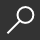## Basic Math and Pre-Algebra : Tutorial and Practice Problems by Carolyn Wheater

Overview -
Idiot's Guides: Basic Math and Pre-Algebra helps readers get up to speed and relearn the primary concepts of mathematics, geometry, and pre-algebra. Content includes basic math operations (addition, subtraction, multiplication, division); word problems; factors and multiples; fractions, decimals, and percents; weights and measures; graphs; statistics and probability; and algebra and geometry basics. A practice problems section is also included to help reinforce the math concepts. This book is ideal for anyone needing a refresher in order to pass entrance exams, such as the GED(R), ASVAB, and Praxis(R).

local_shippingFor DeliveryOn Order. Usually ships in 2-4 weeks
This item is Non-Returnable.
FREE Shipping for Club Members helpNew & Used Marketplace 53 copies from \$3.45

## More About Basic Math and Pre-Algebra by Carolyn Wheater

### Overview

Idiot's Guides: Basic Math and Pre-Algebra helps readers get up to speed and relearn the primary concepts of mathematics, geometry, and pre-algebra. Content includes basic math operations (addition, subtraction, multiplication, division); word problems; factors and multiples; fractions, decimals, and percents; weights and measures; graphs; statistics and probability; and algebra and geometry basics. A practice problems section is also included to help reinforce the math concepts. This book is ideal for anyone needing a refresher in order to pass entrance exams, such as the GED(R), ASVAB, and Praxis(R).

This item is Non-Returnable.

### Details

• ISBN-13: 9781615645046
• ISBN-10: 1615645047
• Publisher: Alpha Books
• Publish Date: August 2014
• Page Count: 368
• Dimensions: 9.25 x 7.75 x 0.75 inches
• Shipping Weight: 1.27 pounds

Series: Idiot's GuidesRelated Categories